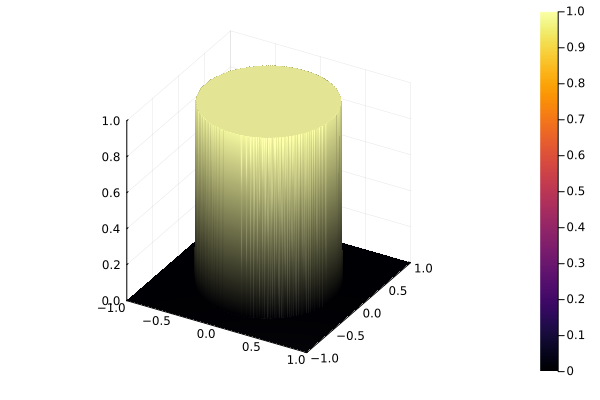# Plotting surface without interpolating at discontinuity

I am attempting to plot a function that has a discontinuity, consider for example,

``````function f(x, y)
if x^2 + y^2 ≤ 1/2
return 1.
else
return 0.
end
end
``````

If I use the `surface` function from `Plots.jl` I obtain the following plot

``````surface(-1:0.01:1, -1:0.01:1, f)
``````Is there a way to plot the function without `surface` automatically interpolating the discontinuity points?

I don’t know of an automatic method, but in general, to obtain the same result, `NaN`s are inserted around the discontinuities to prevent interpolation:

Plots.jl code
``````using Plots; plotlyjs()

function f(x, y, δ)
r = x^2 + y^2
if r ≤ 1/2 - δ
return 1.0
elseif r ≥ 1/2 + δ
return 0.0
end
return NaN
end

δ = 0.01
x = y = -1.0:δ:1.0
Plots.surface(x, y, (x,y)->f(x,y,δ))

heatmap(x, y, (x,y)->f(x,y,δ), ratio=1, lims=(-1,1))
``````
1 Like

Yeah, I thought about this, but in my case the discontinuity points are hard to compute. They are determined by an implicit equation, which I would have to solve with a root finder. I can do that, but it is not that elegant.

Ok, check if this automatic method could work in your case:

``````using Plots; plotlyjs(size=(800,600), dpi=600)

f(x, y) = x^2 + y^2/4 ≤ 1/2 ? 1. : 0.

x = -1.0:0.01:1.0
y = -2.0:0.01:2.0
z = f.(x,y')

ϵ = 0.5
∇x = vcat(diff(z, dims=1), zeros(1, length(y)))
∇y = hcat(diff(z, dims=2), zeros(length(x)))
z[hypot.(∇x, ∇y) .> ϵ] .= NaN

surface(x, y, z', xlabel="x", ylabel="y", zlabel="z")
``````

NB: changed the circular disc to an ellipse for easier debugging

3 Likes

… should also work with GR (or Plots with the default backend):

@jheinen, Plots.jl with gr() backend seems to exhibit several bugs on this example.

Bug on `aspect_ratio=:equal`, which is not applied to the z-axes:

``````surface(x, y, z', ratio=:equal)
``````

Bug on the color scale limits if we set `zlims` to fix the `aspect_ratio` bug above:

``````surface(x, y, z', ratio=:equal, zlims=(-2,2))
``````
1 Like